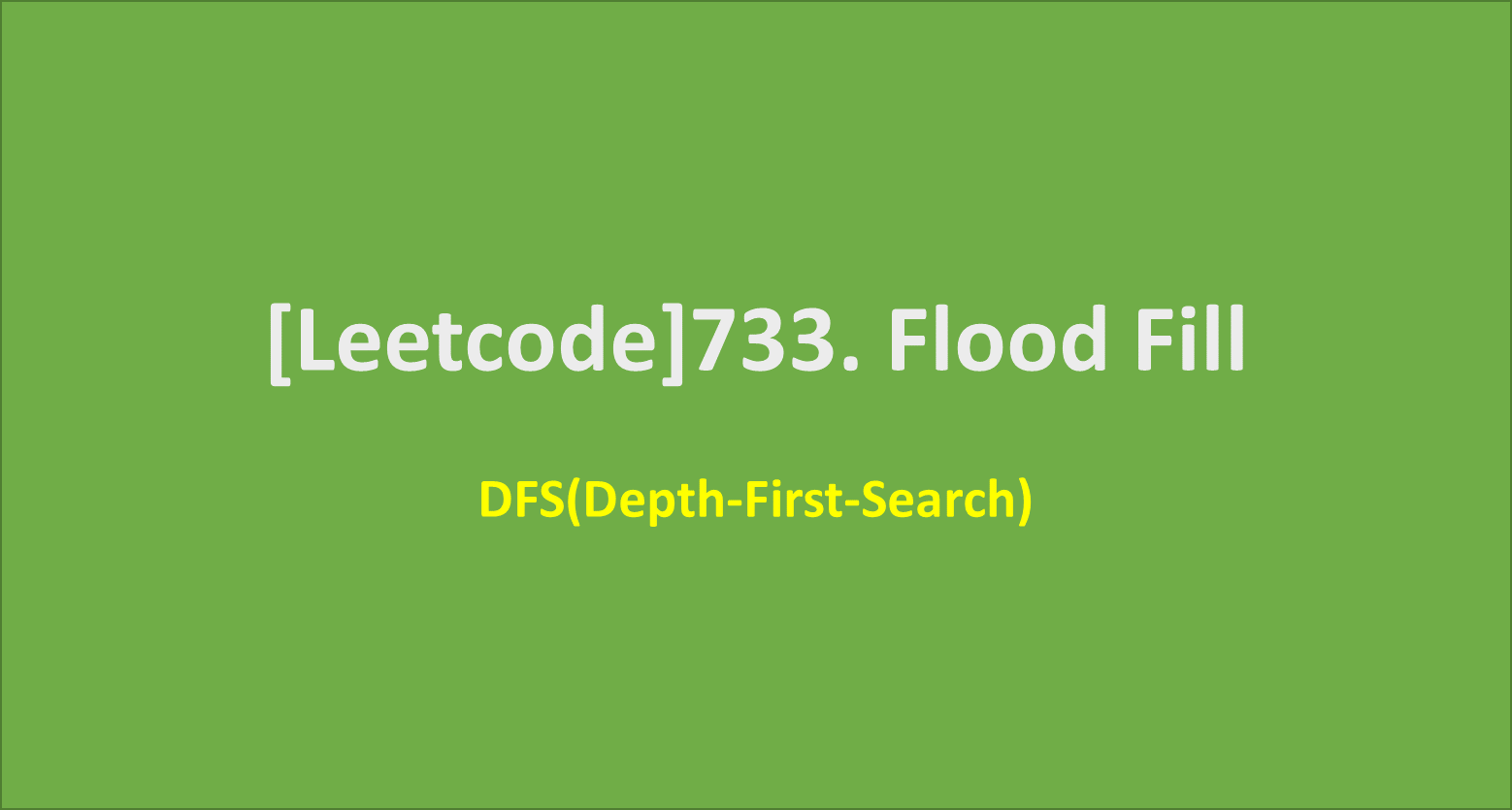# [Leetcode]733. Flood Fill

limelimejiwon·2022년 3월 14일
0

## Python으로 공부하는 코딩테스트

목록 보기
14/67## 📄 Description

An image is represented by an m x n integer grid image where image[i][j] represents the pixel value of the image.

You are also given three integers sr, sc, and newColor. You should perform a flood fill on the image starting from the pixel image[sr][sc].

To perform a flood fill, consider the starting pixel, plus any pixels connected 4-directionally to the starting pixel of the same color as the starting pixel, plus any pixels connected 4-directionally to those pixels (also with the same color), and so on. Replace the color of all of the aforementioned pixels with newColor.

Return the modified image after performing the flood fill.

## 🔨 My Solution

• check the current pixel and paint, plus check the adjacent pixels of the same color.
1. the pixel should be valid one
1. the color of the pixel should be same color
• repeat this painting process through DFS

## 💻 My Submission

class Solution:
def floodFill(self, image: List[List[int]], sr: int, sc: int, newColor: int) -> List[List[int]]:
dr=[-1,1,0,0]
dc=[0,0,-1,1]
r,c=len(image),len(image)

check=[[False]*c for _ in range(r)]
color=image[sr][sc]

def recur(i,j):
check[i][j]=True

if image[i][j]==color:
image[i][j]=newColor

for k in range(4):
nr=i+dr[k]
nc=j+dc[k]
#print(nr,nc)

if nc<0 or nc>=c or nr<0 or nr>=r:
continue

if not check[nr][nc] and image[nr][nc]==color:
recur(nr,nc)
recur(sr,sc)

return image

## 🔎 Complexity

Time Complexity: O(N)
where N is the number of pixels in the image.
Space Complexity: O(N)
the size of the implicit call stack when calling dfs.

## 💊 Clean code

class Solution(object):
def floodFill(self, image, sr, sc, newColor):
R, C = len(image), len(image)
color = image[sr][sc]
if color == newColor: return image
def dfs(r, c):
if image[r][c] == color:
image[r][c] = newColor
if r >= 1: dfs(r-1, c)
if r+1 < R: dfs(r+1, c)
if c >= 1: dfs(r, c-1)
if c+1 < C: dfs(r, c+1)

dfs(sr, sc)
return image

### 💡 How can I improve my solution?

1. Without using dr and dc, we can split the four adjacent position to if condition and dfs each of them.
2. I used check array for checking if the pixel have been already checked, to avoid checking again. And I'm curious if it is efficient for decreasing time..?